﻿ Numerical Investigation of the Baffle Effect on the Hydrodynamic Structure of a Container Partially Filled with Liquid

### Numerical Investigation of the Baffle Effect on the Hydrodynamic Structure of a Container Partially ...

Abdallah Bouabidi, Zied Driss, Mohamed Salah Abid

American Journal of Mechanical Engineering

## Numerical Investigation of the Baffle Effect on the Hydrodynamic Structure of a Container Partially Filled with Liquid

Abdallah Bouabidi1,, Zied Driss1, Mohamed Salah Abid1

1Laboratory of Electro-Mechanic Systems (LASEM), National School of Engineers of Sfax (ENIS), University of Sfax B.P. 1173, km 3.5 Soukra, 3038 Sfax, TUNISIA

### Abstract

Under sloshing loads, pressure on the walls increases significantly in the partially filled container subjected to external excitations. To reduce the pressure value, the use of baffles was developed as a solution for this problem. In this work, we are interested on the investigation of the baffle effect on the hydrodynamic structure of the container. The numerical simulation was performed using the commercial software “Fluent”. To impose a sinusoidal external excitation, a User Defined Function (UDF) was developed. The VOF model was used to simulate the two phase phenomenon. The hydrodynamic parameters such as, velocity fields, vorticity, static pressure, dynamic pressure and turbulence parameters are presented and discussed. The numerical results confirm that the hydrodynamic parameters are affected specially around the baffle. The validation of our numerical model was assured by comparing the numerical results with the experimental one for the case of the unbaffled container.

• Abdallah Bouabidi, Zied Driss, Mohamed Salah Abid. Numerical Investigation of the Baffle Effect on the Hydrodynamic Structure of a Container Partially Filled with Liquid. American Journal of Mechanical Engineering. Vol. 4, No. 3, 2016, pp 112-123. http://pubs.sciepub.com/ajme/4/3/5
• Bouabidi, Abdallah, Zied Driss, and Mohamed Salah Abid. "Numerical Investigation of the Baffle Effect on the Hydrodynamic Structure of a Container Partially Filled with Liquid." American Journal of Mechanical Engineering 4.3 (2016): 112-123.
• Bouabidi, A. , Driss, Z. , & Abid, M. S. (2016). Numerical Investigation of the Baffle Effect on the Hydrodynamic Structure of a Container Partially Filled with Liquid. American Journal of Mechanical Engineering, 4(3), 112-123.
• Bouabidi, Abdallah, Zied Driss, and Mohamed Salah Abid. "Numerical Investigation of the Baffle Effect on the Hydrodynamic Structure of a Container Partially Filled with Liquid." American Journal of Mechanical Engineering 4, no. 3 (2016): 112-123.

 Import into BibTeX Import into EndNote Import into RefMan Import into RefWorks

### 1. Introduction

The sloshing in the partially filled container is a phenomenon of interest in several engineering applications. It generates a significant increase on the pressure value in the container walls. Thus, several research works have been devoted in to investigate the liquid sloshing. Hou et al.  conducted a series of numerical simulations to analyze the sloshing problem. They performed the numerical simulation for the case of single excitation and for the case of multiple coupled excitations. Jung et al.  analyzed the liquid sloshing in a partially filled tank equipped with vertical baffle. They showed that the use of the vertical affects the sloshing in the tank and the pressure decreases significantly on the tanks walls with the baffle use. Bouabidi et al.  analyzed the effect of the vertical baffle height to suppress the sloshing violence in the partially filled container using the CFD code. Their study confirmed that the sloshing decreases with the increase of the baffle height. Singal et al.  developed a CFD analysis of a kerosene fuel container to reduce liquid sloshing using the commercial finite volume package ANSYS FLUENT. They found that the Kerosene liquid interface that the sloshing in the fuel container was significantly reduced with the introduction of baffles in the fuel tank. Jin et al.  studied the sloshing in a container subjected to an external sinusoidal excitation. They investigated the effect of horizontal perforated plate on decreasing the sloshing violence. The study showed that the parameters of the sinusoidal excitation affect the behavior of the sloshing in the container. Also, they found that the use of the perforated baffle can decrease the liquid sloshing. Bouabidi et al.  analyzed of the time step size effect for the numerical simulation of sloshing. They compared their numerical results with the experimental results. They showed that the choice of the optimum time step value leads to a good agreement for the comparison of the numerical and the experimental results. Chen et al.  developed finite difference method to study the sloshing of liquid in containers. They found that for a constant frequency, the sloshing amplitude at the wall increases linearly with Reynolds number. However, the sloshing displacement depends to the frequency for a constant Reynolds number. Mnasri et al.  investigated a two dimensional model based on CFD code simulation of the interaction between the free surface and moving bodies with constant and time dependent velocities. The effect of the baffle in the liquid sloshing phenomena is also interest of many researchers. Liu et al.  studied the effect of the vertical and horizontal baffle in the liquid sloshing in a tank. They showed that sloshing is more reduced using the vertical baffle than using the horizontal baffle. Bouabidi et al.  investigated the liquid sloshing in a battery cell with mixing element partially filled with liquid using the commercial CFD code “Fluent”. They studied the pumping phenomena under pressure variation in the tank. A test bench was developed to investigate the sloshing experimentally in the battery cell. The good comparison between the numerical and the experimental results validated the numerical model. Sanapala et al.  used the CFD technique to simulate the dynamics of liquid sloshing and its control in a storage container for spent fuel applications. They developed a systematic numerical simulations in a container subjected to seismic excitations. To suppress the sloshing loads, they tested the efficient of two baffle types; the ring and the vertical baffle. Bouabidi et al.  carried out a series of experiments to study a hydrostatic pump in a partially filled container. To understand the source of the hydrostatic pump, they performed a CFD simulation to predict the static pressure distribution generated in the container under sloshing loads. Jung et al.  analyzed the liquid sloshing in a partially filled tank equipped with vertical baffle. They showed that the use of the vertical affects the sloshing in the tank and the pressure decreases significantly on the tanks walls with the baffle use. Bouabidi et al.  conducted a series of numerical simulations to investigate the effect of the external excitation frequency. They used the VOF (volume of fluid) model to resolve the two phase problem. The study confirms that the sloshing behavior depends on the frequency value. In fact, the sloshing loads increase with the frequency increase.

In this paper, we propose to examine the liquid sloshing in a partially filled container subjected to an external sinusoidal excitation. Particularly, we are interested on the study of the baffle effect.

### 2. Geometry Configuration

The geometrical arrangement is similar to Panigrahy et al.  application. As shown in Figure 1, the considered 2d geometry is defined by the length L=0.6 m and the height equal to H=0.6 m. The container is partially filled with liquid with the height h=0.1 m. In this work, we are interested on the study of the baffle effect. The container is equipped with different baffle heights equals to; b=50 mm, b=75 mm and b=100 mm. The baffle are defined by the ration equal to r=b/h.Download asVeiw figureFigures index
Figure 1. Geometrical arrangement

### 3. Numerical Model

The mathematical model is given by the Navier-Stokes equations defined as follows :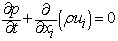(1)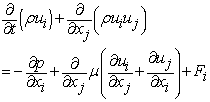(2)

Where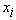are Cartesian coordinates,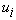are the corresponding velocity components, p is the pressure,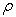is the density,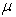is the viscosity and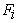is the external body force.

The external body force is Fi written as follows: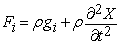(3)

Where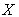represents the external sinusoidal excitation.

The turbulence kinetic energy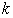and its rate of dissipation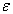are obtained from the following transport equations :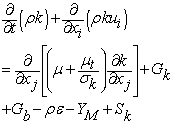(4)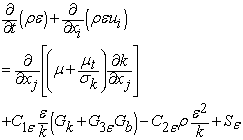(5)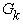and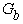are the generation of turbulent kinetic energy (kg.m-1.s-3) respectively due to the mean velocity gradients and buoyancy.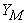represents the contribution of the fluctuating dilatation in compressible turbulence to the overall dissipation rate. The turbulent viscosity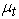(Pa.s) is given by: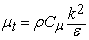(6)

Where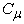is a constant.

The default values of the constants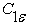,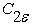,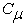,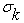and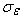are presented in Table 1.

#### Table 1. model constantsDownload asPowerPoint Slide

Veiw figure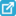View current table in a new window

The commercial CFD code “Fluent” is used to develop the simulation of the sloshing problem in the partially filled tank.

In “Fluent”, two models are available for simulations where two or more fluids are presented. The Volume of Fluid (VOF) model is designed for two or more immiscible fluids, where the position of the interface between the fluids is of interest. The Eulerian model is designed for two or more interpenetrating fluids. Whereas, the Eulerian model makes use of multiple momentum equations to describe the individual fluids, the VOF model does not. In the VOF model, a single set of momentum equations is shared by the fluids, and the volume fraction of each of the fluids is tracked throughout the domain. Applications of the VOF model include the prediction of jet break-up, the motion of large bubbles in a liquid, the motion of liquid after a dam break, or the steady or transient tracking of any liquid-gas interface. The Eulerian multiphase model, on the other hand, is best suited for applications involving slurries or liquid-liquid and liquid gas mixtures involving small bubbles. Thus, in our application the model VOF is used.

The free surface is defined as the zero level set of a level-set function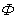: in air, is set to be positive and in water, to be a negative function as follows: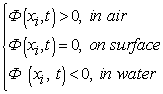(7)

Differentiating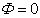with respect to time, a transport equation is derived to describe the free surface motion in the form:(8)

Where ui is the local fluid velocity and, at any time, moving the interface is equivalent to updatingby solving equation (8).

### 4. Numerical results

4.1. Free surface evolution

Figure 2, Figure 3, Figure 4 and Figure 5 present respectively the free surface deformation at t=1 s, t=2 s , t=3 s and t=4 s for different tanks design, the unbaffled container and the baffled container with different baffle heights equals to; b=50 mm, b=75 mm and b=100 mm. The numerical results indicated that the sloshing behavior is affected by the baffle. In fact, the liquid sloshing in the container equipped with baffle is different to the unbaffled tank. The blockage effect of the baffle increases with the increase of the baffle height. Over time, the liquid motion occurs in the partially filled container under the external excitation effect. For the first half period time, the liquid moves from the right to the left. Whereas, the liquid motion occurs form the left to the right. The presented result showed that the used baffle reduces the sloshing violence. Thus, the pressure on the container walls decreases significantly.Download asVeiw figureFigures index
Figure 2. Free surface deformation at t=1 sDownload asVeiw figureFigures index
Figure 3. Free surface deformation at t=2 sDownload asVeiw figureFigures index
Figure 4. Free surface deformation at t=3 sDownload asVeiw figureFigures index
Figure 5. Free surface deformation at t=4 sDownload asVeiw figureFigures index
Figure 6. Velocity field at t=1 sDownload asVeiw figureFigures index
Figure 7. Velocity field at t=2 sDownload asVeiw figureFigures index
Figure 8. Velocity field at t=3 sDownload asVeiw figureFigures index
Figure 9. Velocity field at t=4 s
4.2. Velocity Field

Figure 6, Figure 7, Figure 8 and Figure 9 depict the velocity fields for the different considered configurations respectively at t=1 s, t=2 s, t=3 s and t=4 s. According to these results, it has been noted that the liquid motion generates the appearance of velocity fields in the different considered tanks; without baffle and baffled with different baffle height. For the different considered cases, it has been observed the appearance of recirculation zones in the whole volume of the tank. The velocity field are parallel to the walls close the walls. In another hand, the location of the maximum value changes over time. For the baffled tank, a significant recirculation zone appears around the baffle. A very important recirculation zone has been observed for the case of the baffle height equal to b= 50 mm. in addition, it has been observed that the velocity value varies from configuration to another at each instant. Thus, the use of the baffle affects the velocity value distribution in the container.

4.3. Magnitude Velocity

The distribution of the magnitude velocity caused by the liquid sloshing at different probes are depicted in Figure 10, Figure 11, Figure 12 and Figure 13 for the different considered configurations; the unbaffled container and the tanks with vertical baffle heights equal to b=50 mm, b=75mm, and b=100 mm. For the tanks without baffle, the highest value of velocity is located between the free surface and the side up wall and near the both left side wall. However, with vertical baffle heights equals to b=50 mm and b=100 mm, the highest value of magnitude velocity is located at the free surface and above the baffle. These results confirm that the used baffle affects the hydrodynamic structure of the tank, specially the magnitude velocity. In addition, it has been noted that the baffle effect depends on its height.

4.4. Static Pressure

The distribution of the static pressure caused by the liquid sloshing is shown in Figure 14, Figure 15, Figure 16 and Figure 17 for the different considered container, without baffle and with different baffle heights. According to these results, the compression zones and the depression zones are occurred in the whole volume of the container as a result of the liquid motion. The location of these zones depends on the external excitation direction. During the first half period time of the external excitation, the liquid moves from the right to the left and the compression zones appear in the left bottom corner. However, the compression zones appear in the right bottom corner during the second half period time. For the unbaffled tank, the static pressure is totally exerted on the walls. However, the pressure is exerted on the container walls and on the baffle for the case of the baffled tank. In another hand, it has been noted that the maximum pressure value varies from configuration to another. For the different considered instants, the maximum value of is observed for the unbaffled tank, whereas the minimum value appears for the baffled tank with r=075. Over time, the maximum static pressure value reaches different values for all the considered geometries in this study. In fact, the maximum static pressure value reaches its maximum value at t=1 s and t=3 s, whereas it reaches its minimum value at t=2 s and t=4 s.Download asVeiw figureFigures index
Figure 10. Distribution of the magnitude velocity at t=1 sDownload asVeiw figureFigures index
Figure 11. Distribution of the magnitude velocity at t=2 sDownload asVeiw figureFigures index
Figure 12. Distribution of the magnitude velocity at t=3 sDownload asVeiw figureFigures index
Figure 13. Distribution of the magnitude velocity at t=4 sDownload asVeiw figureFigures index
Figure 14. Distribution of the static pressure at t=1 sDownload asVeiw figureFigures index
Figure 15. Distribution of the static pressure at t=2 sDownload asVeiw figureFigures index
Figure 16. Distribution of the static pressure at t=3 sDownload asVeiw figureFigures index
Figure 17. Distribution of the static pressure at t=4 s

### 5. Comparison with Experimental Results

Figure 18 depicts the pressure evolution over time at the point P1 for the different considered configurations of the container and the experimental results of Panigrahy et al. . For the case of the container partially filled with liquid without baffle, our numerical results are compared with the experimental results of Panigrahy et al.  and a good agreement has been observed. In addition, it has been noted that the pressure varies periodically over time for the unbaffled and the baffled tank. These results are expected since the container is subjected to an external sinusoidal excitation. In another hand, it has been observed that the use of the vertical baffle affects the static pressure profile and value. All the static pressure profiles vary periodically for the considered tank. However, it the period profile varies from configuration to another. In fact, the maximum period value is observed for the baffled tank with r=0.75 and the minimum period value has been observed for the case of the unbaflled tank.Download asVeiw figureFigures index
Figure 18. Comparison with experimental results

### Acknowledgement

The research is conducted in an MOBIDOC thesis funded by the European Union (EU) under the program PASRI (Projet d’Appui au Système de Recherche et de l’Innovation).

### References

  L. Hou, F.A. Li, Numerical Study of Liquid Sloshing in a Two-dimensional Tank under External Excitations. Journal of Marine Science and Application, 11, 305-310, 2012.In article  J.H. Jung, H.S. Yoon, C.Y. Lee, S.C. Shin, Effect of the vertical baffle height on the liquid sloshing in a three dimensional rectangular tank, Ocean Engineering, 44, 79-89, 2012.In article  A. Bouabidi, Z. Driss, M.S. Abid, Vertical Baffles Height Effect On Liquid Sloshing In An Accelerating Rectangular Tank, International Journal of Mechanics and Applications, 3, 105-116, 2013.In article  V. Singal, J. Bajaj, N. Awalgaonkar, S. Tibdewal, CFD Analysis of a Kerosene Fuel Tank to Reduce Liquid Sloshing, Procedia Engineering, 69, 1365-1371, 2014.In article  H. Jin, Y. Liu, J.H. Li, Experimental study on sloshing in a tank with an inner horizontal perforated plate, Ocean Engineering, 82, 75-84, 2014.In article  A. Bouabidi, Z. Driss, M.S. Abid, Time Step Size Effect on the Liquid Sloshing Phenomena, International Journal of Fluid Mechanics & Thermal Sciences, 1, 8-13 , 2015.In article  B. Chen, R. Nokes, Time-independent finite difference analysis of fully non-linear and viscous fluid sloshing in a rectangular tank, Journal of Computational Physics, 209, 47-81, 2005.In article  C. Mnasri, Z. Hafsia, M. Omri, K. Maalel, A moving grid model for simulation of free surface behavior induced by horizontal cylinders exit and entry, Engineering Applications of Computational Fluid Mechanics, 4, 260-275, 2010.In article  D. Liu, P. Lin, Three dimensional liquid sloshing in a tank with bafﬂes, Ocean Engineering, 36, 202-212, 2009.In article  A. Bouabidi, Z. Driss, M. Kossentini, M.S. Abid, Numerical and Experimental Investigation of the Hydrostatic Pump in a Battery Cell with Mixing Element, Arabian Journal for Science and Engineering, 2016, 41, 1595-1608.In article  V.S. Sanapala, K. Velusamy, B.S.V. Patnaik, CFD simulations on the dynamics of liquid sloshing and its control in a storage tank for spent fuel applications, Annals of Nuclear, Energy, 94, 494-509, 2016.In article  A. Bouabidi, Z. Driss, M.S. Abid, Study of hydrostatic pump created under liquid sloshing in a rectangular tank subjected to external excitation, International Journal of Applied Mechanics, 08, 1-15, 2016.In article  M. Jiang, B. Ren, G. Wang, Y. Wang, Laboratory investigation of the hydroelastic effect on liquid sloshing in rectangular tanks, Journal of Hydrodynamics, 26, 751-761, 2014.In article  A. Bouabidi, Z. Driss, N. Cherif, M.S. Abid, Computational investigation of the external excitation frequency effect on liquid sloshing phenomenon, WSEAS Transactions on Fluid Mechanics, 11, 1-9, 2016.In article  P. K. Panigrahy, , P. K. Saha, U. K. Maity, Experimental studies on sloshing behavior due to horizontal movement of liquids in baffled tanks, Ocean Engineering, 36, 213-222, 2009.In article  Z. Driss, M.S. Abid, Use of the Navier-Stokes Equations to Study of the Flow Generated by Turbines Impellers, Navier-Stokes Equations: Properties, Description and Applications, Chapter 3, pp. 51-138, 2012.In article